# How To Change The Order Of DataFrame Columns?

A DataFrame is the primary data structure of the Pandas library and is commonly used for storing and working with tabular data. Pandas is a Python library used for data analytics.

A common operation that could be performed on such data is changing the order of DataFrame columns to make it easy to work with and increase comprehensibility.

To start working with Pandas, we first need to import it:

``import pandas as pd``

## Running Example: Create a DataFrame in Pandas

Consider the following DataFrame containing 3 students with names A, B, and C and their corresponding marks (out of 10) for two subjects, Mathematics and Physics.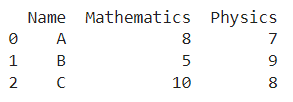Code snippet for generating the above DataFrame:

``````import pandas as pd
# Dictionary for our data
data = {'Name' : ['A', 'B', 'C'], 'Mathematics' : [8, 5, 10], 'Physics' : [7, 9, 8]}

# DataFrame for the dictionary
df = pd.DataFrame(data)

# Printing the DataFrame
print(df)``````

Here, data is a dictionary, we created to initialize the DataFrame. For this, we use the DataFrame() function/constructor of the Pandas library which takes the dictionary as an argument and returns the required DataFrame.

Let us also calculate the corresponding total marks for each student: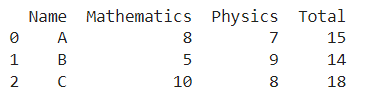Code snippet for generating the above DataFrame:

### Python 3 Code:

``````# Adding a new column named 'Total'

df['Total'] = df['Mathematics'] + df['Physics']

# Printing the DataFrame with new column 'Total'

print(df)``````

Let’s say, we need to add another subject’s marks to this DataFrame, say History and recompute the total, the resulting DataFrame and corresponding code snippet would look as follows :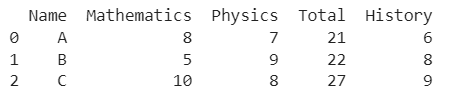## Python Examples: Add a new Column in Pandas Dataframe

``````# Adding a new column named 'History'
df['History'] = [6,8,9]

# Recomputing Total Marks
df['Total'] = df['Mathematics'] + df['Physics'] + df['History']

# Printing the DataFrame
print(df)``````

Although the operation we have performed seems to have worked just fine, doesn’t the DataFrame look a bit odd when we view it from a layman’s perspective? Ideally the Total column should be the last one and the resulting DataFrame should be as follows :Let’s look at different ways to rectify this, along with the time required to run the corresponding code snippets :

We shall use the time library to keep track of time. To import it, use the following code statement:

``import time``

## Manually ordering the columns using column names in pandas dataframe

In this method, we manually reorder the columns using specific column names. As you might have realized, this method becomes quite tedious when the number of columns to be rearranged is pretty large, with complex column names. There is greater scope for error in such situations. We use time.time() to keep track of the time.

``````t0 = time.time()

# Creating a new DataFrame df1 with correct order of columns
df1 = df[['Name', 'Mathematics', 'Physics', 'History', 'Total']]

# Printing the DataFrame df1
print(df1)

# Printing time elapsed
print("\nTime elapsed = ", time.time() - t0, " seconds")``````

Output :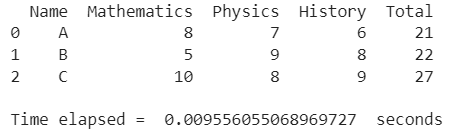## 2. Maintaining the order in a separate list

In this method, we maintain the column-wise order in a separate list. Here the list becomes [0,1,2,4,3], the order being 0-indexed.

This method helps eliminate possible chances of error due to complex column names but if the number of columns is large, it is still a tedious way to change column order.

We use time.time() to keep track of the time.

``````t0 = time.time()

# List containing order
order = [0,1,2,4,3]

# Creating a new DataFrame df2 with correct order of columns
df2 = df[[df.columns[i] for i in order]]

# Printing the DataFrame df2
print(df2)

# Printing time elapsed
print("\nTime elapsed = ", time.time() - t0, " seconds")``````

Output :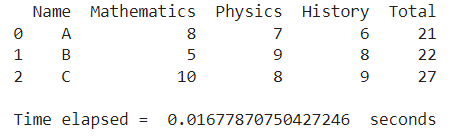## 3. Change Dataframe Order using a for loop in Pandas

In this method, we let all columns except Total remain in the same order and shift Total towards the end.

This method works great for this case where we need to change the position of only one column, Total, but we can see the complexity rising if the number of columns whose position needs to be changed increases. We use time.time() to keep track of the time.

``````t0 = time.time()

# Creating a new DataFrame df3 with correct order of columns
df3 = df[[col for col in df.columns if col != 'Total'] + ['Total']]

# Printing the DataFrame df3
print(df3)

# Printing time elapsed
print("\nTime elapsed = ", time.time() - t0, " seconds")``````

Output :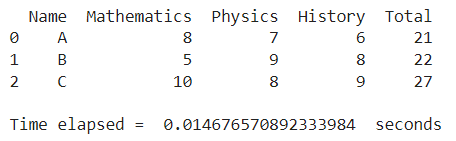## 4. Change Dataframe Order using clever indexing in Pandas

In this method, we first take all columns except the last two, i.e Total and History, after which we take the History column and then the Total column.

This method could also lead to complications in case the number of columns whose order needs to be changed is large. We use time.time() to keep track of the time.

The cols[:-2] represents all columns except the last two, cols[-1] represents the last column, and cols[-2] represents the second-last column.

``````t0 = time.time()

# Creating a new DataFrame df4 with correct order of columns
cols = list(df)
cols = cols[:-2] + [cols[-1]] + [cols[-2]]
df4 = df[cols]

# Printing the DataFrame df4
print(df4)

# Printing time elapsed
print("\nTime elapsed = ", time.time() - t0, " seconds")``````

Output :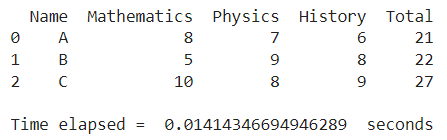## 5. Change Dataframe Order using reindex() function in Pandas

In the reindex() function, the first argument is a list specifying the new column order and setting the second argument, axis as 1 specifies that the reindexing has to be done column-wise. We use time.time() to keep track of the time.

``````t0 = time.time()

# Creating a new DataFrame df5 with correct order of columns
df5 = df.reindex(['Name', 'Mathematics', 'Physics', 'History', 'Total'], axis = 1)

# Printing the DataFrame df5
print(df5)

# Printing time elapsed
print("\nTime elapsed = ", time.time() - t0, " seconds")``````

Output :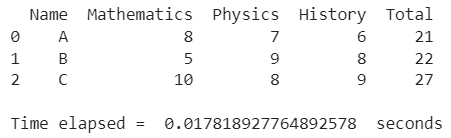## 6. Change Dataframe Order using the  .loc property in Pandas

In this method, we use the .loc property of a DataFrame. The first argument having a colon(:) specifies that we need all the rows of the original DataFrame and the second argument mentions the column order, using column names. We use time.time() to keep track of the time.

``````t0 = time.time()

# Creating a new DataFrame df6 with correct order of columns
df6 = df.loc[:, ['Name', 'Mathematics', 'Physics', 'History', 'Total']]

# Printing the DataFrame df6
print(df6)

# Printing time elapsed
print("\nTime elapsed = ", time.time() - t0, " seconds")``````

Output :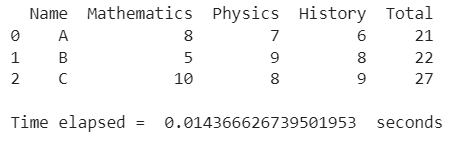## 7. Change Dataframe Order using the  .iloc property in Pandas

In this method, we use the .iloc property of a DataFrame. The first argument having a colon(:) specifies that we need all the rows of the original DataFrame and the second argument mentions the column order, using column indices. We use time.time() to keep track of the time.

``````t0 = time.time()

# Creating a new DataFrame df7 with correct order of columns
df7 = df.iloc[:, [0,1,2,4,3]]

# Printing the DataFrame df7
print(df7)

# Printing time elapsed
print("\nTime elapsed = ", time.time() - t0, " seconds")``````

Output :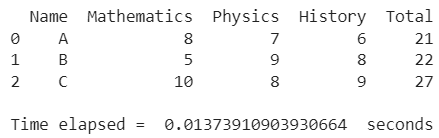## Conclusion

In this topic, we have learned how to change the order of columns in a Pandas DataFrame, following a running example of test scores of students in different subjects, thus giving us an intuition of how this concept could be applied in real-world situations. Feel free to reach out to [email protected] in case of any suggestions.

Trending